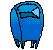Elementary Algebra Assignment #36Solve the following systems by substitution:1. 2. 3. 2x + y = 6 y = x - 3 6x - 4y = 34 y = 3x - 4 4x - y = 4 y = 2x 4.  5. x - 5y = -1 x = 2y - 4 5x + 2y = 15 y = 3x + 2email